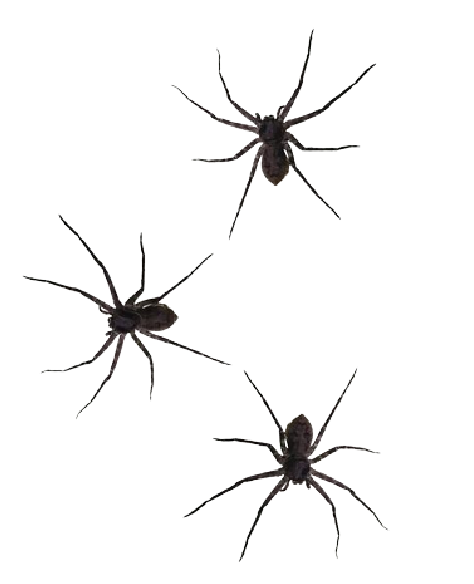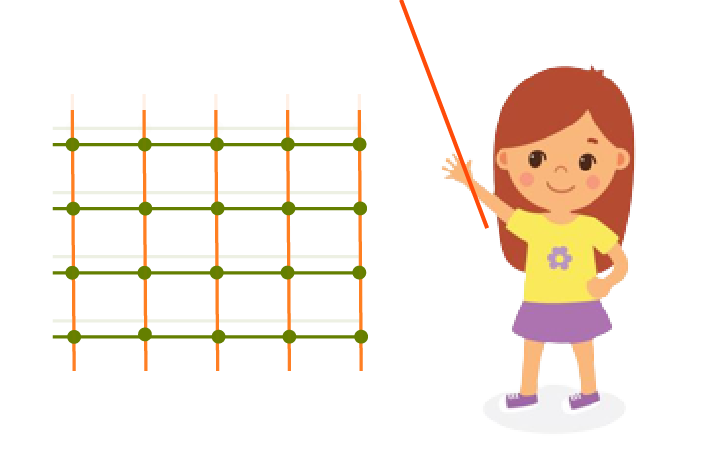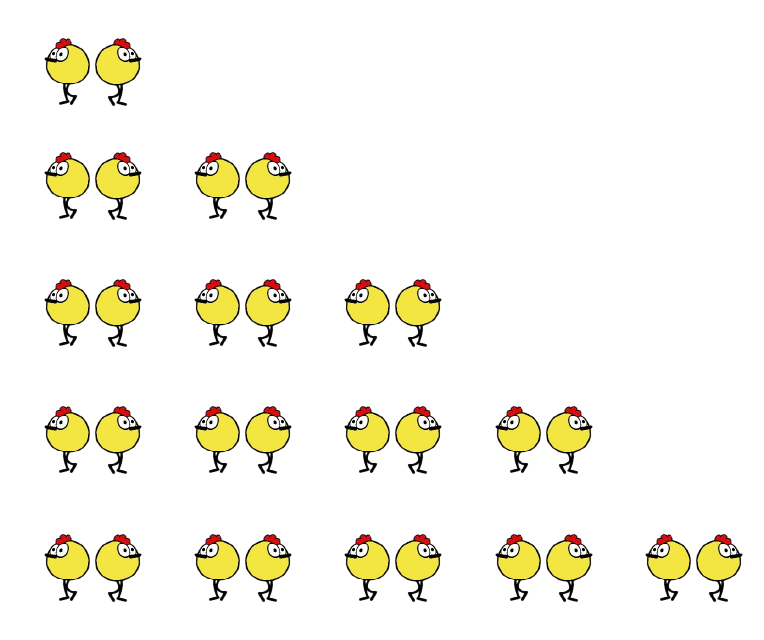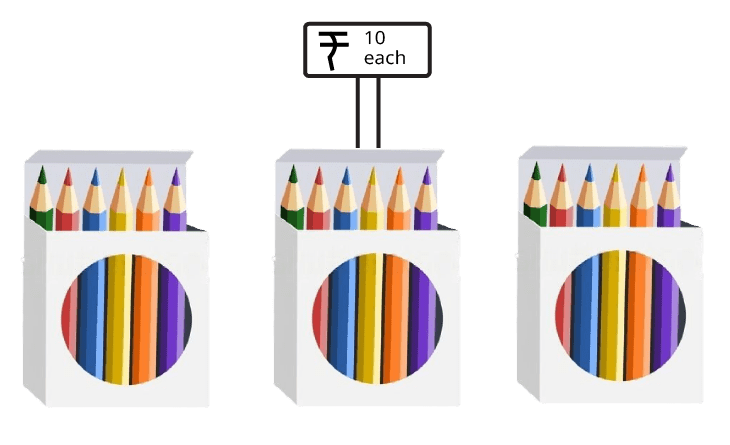Courses
Courses for Kids
Free study material
Free LIVE classes
More

# How Many Times Class 3 Notes CBSE Maths Chapter 9 [Free PDF Download]## Learning Multiplication Made Easy with CBSE Class 3 Maths Chapter 9 Revision Notes

Learning multiplication has been made easier with How Many Times revision notes composed by the top subject experts of Vedantu. All the conceptual portions of this chapter have been explained for simplification following the CBSE standards.

Class 3 students will enjoy learning the tables of single digits through the story-based questions using animals. They will also grasp the concept explained using examples much more easily and can prepare for the exams. Download the revision notes for Chapter 9 how many times for free and use them as reference.

Also, check CBSE Class 3 Maths revision notes for other chapters:

Last updated date: 02nd Jun 2023
Total views: 110.4k
Views today: 0.66k

## How Many Times?

• How many means is the number or counting of anything like there are four chairs, there are two apples.

• Then what is meant by time is simply multiplication or it is repeating about anything.

Solved example 1:  The almirah has 5 shelves. There are 6 books on each shelf. How many books are in the almirah?

Ans: Given, Number of books on each shelf =6

And the total number of shelves = 5

Then total books in almirah = 5 times 6 = 5 × 6 = 30 books.

Hence the total number of books in the almirah = 30.

Solved example 2: Three pens were given to each student in the class. If there were 34 students, How many pens were given in all?

Ans: Total students in class = 34

Each student gets 3 pens.

So, total number of pens given in class = 34 times 3 = 34 ×   3

Hence total pens given in class = 102.

## Leggy Animal

• Animals having long and attractive legs are known as leggy animals.

• There are different numbers of legs for different animals.

• Examples: Four-legged animals, eight legs animals etc. Goats are four-legged animals and octopuses have eight legs.

Solved example 3:  Octopus has 8 legs, then how many legs altogether do 7 octopuses have?

Ans: Given, the Number of legs in one octopus = 8.

The number of octopuses = 7.

Total number of legs in 7 octopuses = 8 times 7 = 8 ×  7 =56.

Hence the number of legs in 7 octopuses = 56.

## How to Find Numbers by Counting• By counting, we can say there are 3 spiders.

Solved Example 4: If a spider has 8 legs, So how many total legs for 3 spiders?

Ans: Here, one spider has 8 legs.

Total number of spiders = 3

Then, the total number of legs for 3 spiders = (8 + 8 + 8)

= 3 x 8 = 24

Therefore, the total number of legs for 3 spiders is 24.

## How to Find Numbers Without Counting

• Here we find the number of flowers in the flower bed without counting them one by one.

• The flower bed has 4 columns and each column has 6 flowers so the total number of flowers will be 4 times 6.

• The total number of flowers in the flower bed = $4\times 6= 24$ flowers.

## Jumping the Number

• 3 Steps each time: In every step, the number is increased by 3.

• 4 Steps each time In every step the number is increased by 4.

• 7 Steps each time In every step the number is increased by 7.

Solved Example 5: Find the missing number in 100, 104, 108, ……., 116, 120.

Ans: The difference between 104 and 100 is 4, likewise between 108 and 104 is 4. So, we can say that in every step the number is increased by 4. Therefore the pattern is the difference between two consecutive numbers is 4 and hence the next number after 108 is ( 108 + 4 ) = 112.

Therefore the required pattern is

100 , 104 , 108 , 112, 116 , 120.

## Stick Play

• In this play, we take some sticks and arrange them horizontally and vertically then we figure how many times sticks are crossing each other.

• Also, we can make tables by using sticks.Sticky Play

• Here, 5 Sticks vertically and 4 sticks horizontally are placed and they are crossing each other 4 times 5.

Solved Example 6: How many different arrangements are possible for 24 sticks.

Ans: To find possible stick arrangements in 24 sticks. First, you need to find all possible multiples of 24: 2, 4, 6, 8, 12, 24.

Possible arrangements 212, 38, 64, 122, 83, 46.

## Multiplication Table of 2

 One time two 1×2 2 Two times two 2×2 4 Three times two 3×2 6 Four times two 4×2 8 Five times two 5×2 10 Six times two 6×2 12 Seven times two 7×2 14 Eight times two 8×2 16 Nine times two 9×2 18 Ten times two 10×2 20

## Practice Question

Q1. Sonu bought 5 packets of biscuits. Each packet has 6 biscuits. How many biscuits did Ramu buy?

Q2. In the figure below, find how many 2 in each row.Arrangement of Birds

Q3. How much do 3 pencil boxes cost in rupees?Pencil Box

Ans: 1. 5 times 6 Sonu brought 30 biscuits.

Ans: 2.

Row 1 one time 2.

Row 2 two times 2.

Row 3 three times 2.

Row 4 four times 2.

Row 5 five times 2.

Ans: 3.

The cost of 3 pencil boxes will be 30 rupees.

Given that each pencil box cost = Rs. 10.

So, the Cost for 3 pencil boxes = 3 times Rs. 10.

Therefore the cost of 3 pencil boxes will be 30 rupees.

### Importance of CBSE Class 3 Maths Chapter 9 How Many Times

• This unique chapter has been formulated using stories and descriptions of animals. Children will get to practice the basic concepts of multiplication and leggy animals.

• They will also learn how to count the flowers and other objects in a stack by using the method of multiplication. In fact, the concepts explained by the experts will also help them realise how repetitive addition can be done using a multiplication method.

• On proceeding further, the chapter will also explain how to multiply the same numbers mentioned in a sum with its repetition to get an answer.

• The next sections and exercises will explain to students how the arithmetic problems can be solved using the same concepts. In these sections, they will learn how to deduce the numbers from the given information and operate mathematically to find the right answers.

• In this chapter, students will learn how to perform 3rd Class multiplication at this level by following the technical words mentioned in the problems.

### Benefits of Vedantu’s How Many Times Class 3 Worksheets and Revision Notes

By using the revision notes and worksheets of this chapter, you will gain the following benefits.

1. Explanation of repetitive addition with multiplication

2. Solving and understanding the question patterns emphasised by the CBSE board

3. Learning how to relate and utilise the tables of single-digit numbers easily

4. Understanding the simplest methods of solving questions by following the steps explained by the subject experts

5. Precise explanation of all the conceptual sections in a comprehensible language for quick revision before an exam

6.  Availing of a practice platform by solving worksheets formulated by experts

7. Evaluation of your conceptual knowledge and mathematical skills based on this chapter.

Download the Class 3 Maths Chapter 9 PDF and add it to your study material. Finish studying this chapter in accordance with school and revise it using these notes. Solve the worksheets once you are done with the chapter exercises.

### Solving How Many Times Class 3 Made Easier

Download the NCERT solutions for Class 3 Maths Chapter 9 for free along with the revision notes. Use both these files to revise the chapters and prepare for the exams. Stay ahead of the competition by using the techniques shown by the expert academicians to solve multiplication questions.

## FAQs on How Many Times Class 3 Notes CBSE Maths Chapter 9 [Free PDF Download]

1. How can I understand the repetitive addition concept with tables?

Follow the explanation given in the revision notes. Check how the tables of single-digit numbers are used to solve the questions. Focus on how the numbers are picked and multiplied to get answers for repetitive additions.

2. How can I practice questions for Chapter 9?

Get hold of the worksheets prepared by the experts. Solve the questions and match your answers. Practice the sums of these sheets apart from the exercise questions.

3. What is multiplication?

It is a mathematical operation that reduces repetitive addition into simpler and smaller steps using tables of numbers.